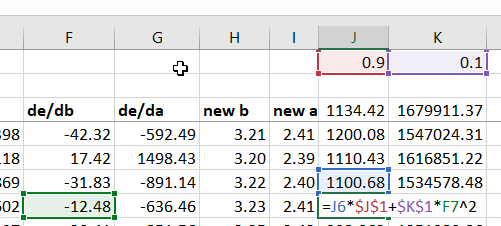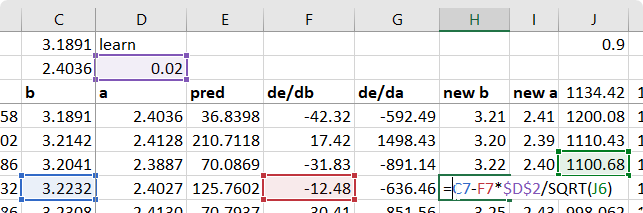# Lesson 5 - RMSProp calculations doubts (graddesc.xlsm)

Hi,

I’ve some doubts about how rmsprop it is calculated in the spreadsheet graddesc.xlsm and in the formula. In the spreadsheet I see calculations like this:``````v_prev(J6) = v_prev_prev(J5)*alpha(J1) + (1-alpha)(K1)* g_prev^2
new_b(F7) = old_b(C7) - lr(D2) * de/deb(F7)/sqrt(v_prev(J6))
``````

But when I look in some articles the square is not from the previous of “exponentially average of the square of gradients”, but of the current calculated. I recalculate with the second option and it got a worse result than what Jeremy show is the video.

Is it matter? It’s maybe I don’t understand the calculations.

Best regards

1 Like

Interesting observation, I hadn’t noticed this. If we use the current gradient as is described here http://ruder.io/optimizing-gradient-descent/index.html#rmsprop, we should be dividing by sqrt(J7) to compute H7. I don’t think it should matter much.

Edit: In the Adam sheet, you’ll see that Jeremy uses the current square of the gradient.

1 Like

I am fairly sure you are correct in your observation. The only time it is noticeable is when you clear out the values and initialize those values to 0 (J3 and K3). If you do this, it matters. Thanks for pointing it out. I came to ask the same question as I am finally starting to get to this level of comprehension.1 Like